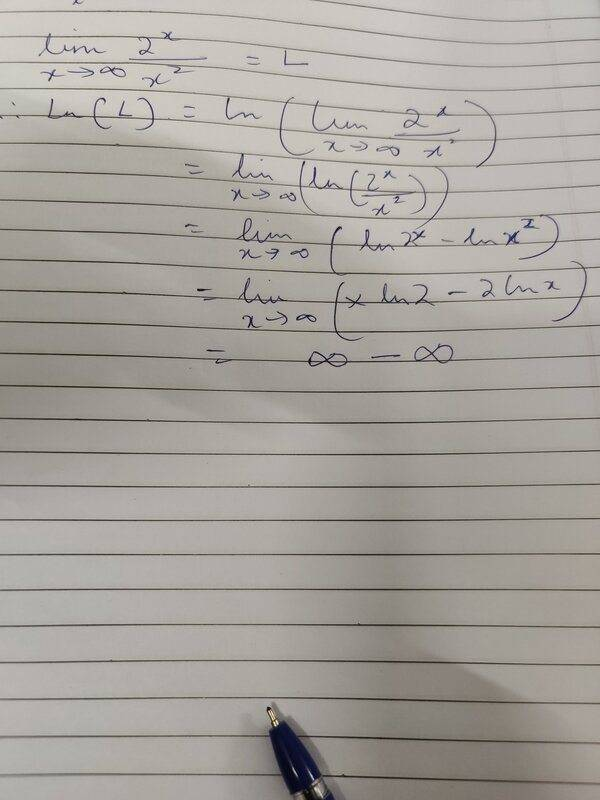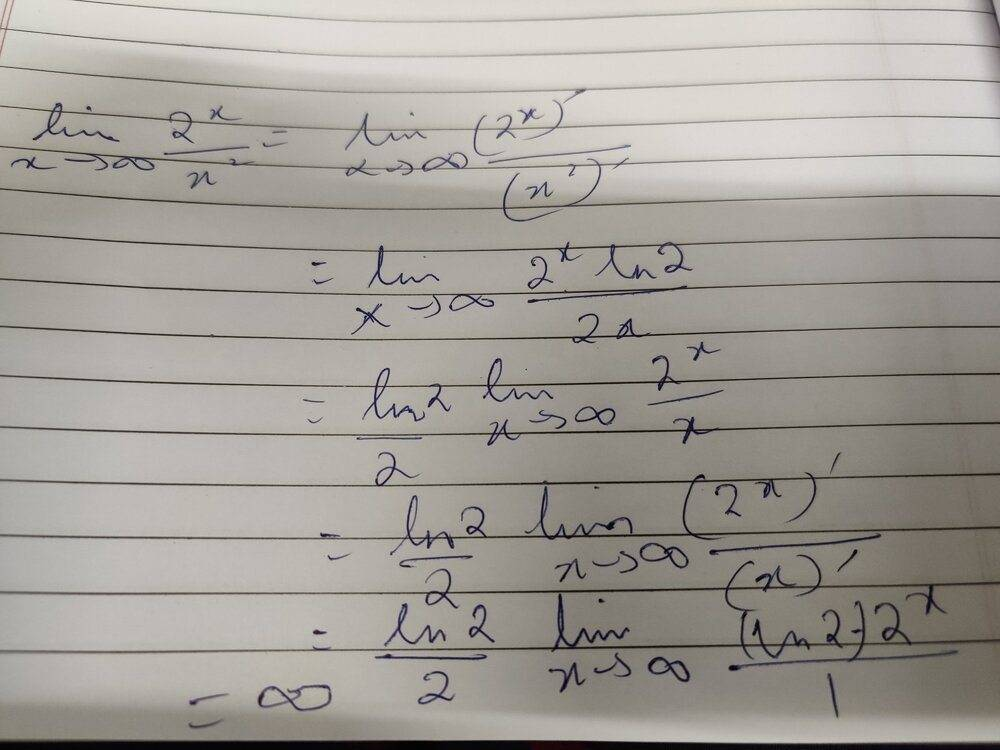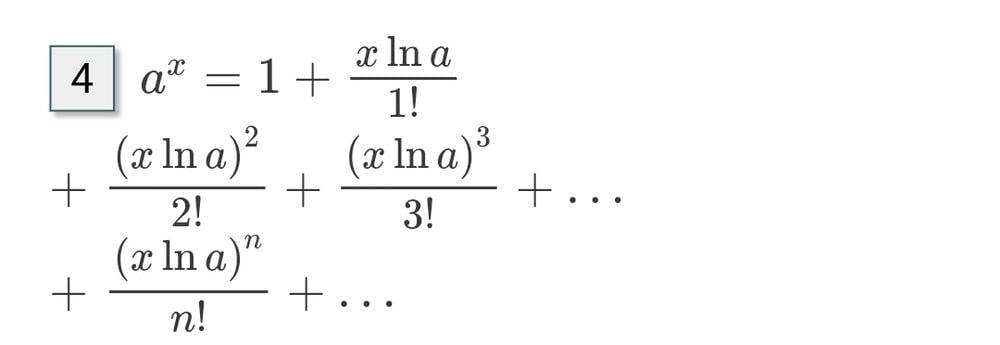# Determine the limit of 2^x/x^2 as x approaches infinity....

• vcsharp2003
In summary: M## whenever x>C.##Since ##\lim_{x\to \infty } \frac{2^{x}}{x^{2} }>M## for all ##x>C##, it follows that ##\lim_{x\to \infty } \dfrac{2^{x}}{x^{2} }>M## whenever x>C.##So, the sequence goes to infinity.Use logarithms? Use L'Hopital's rule?Use logarithms.Use logarithms

#### vcsharp2003

Homework Statement
Determine limit of following expression as x approaches +infinity
Relevant Equations
Limits of complex functions
How would I determine the following limit without substitution of large values of x to see what value is approached by the complex function?
## \lim_{x \rightarrow +\infty} {\dfrac {2^{x}} {x^{2} } } ## where ## x\in \mathbb{R}##

Last edited:
Use logarithms? Use L'Hopital's rule?

•vcsharp2003
PeroK said:
Use logarithms?
I would take log of the complex expression which would still end up with infinity - infinity. Right?

vcsharp2003 said:
I would take log of the complex expression which would still end up with infinity - infinity. Right?
Let's see!

PeroK said:
Let's see!Doesn't that help?

PeroK said:
Use L'Hopital's rule?
When I use this approach, I get a clear answer.PeroK said:
Doesn't that help?
Infinity minus infinity doesn't get me a clear answer.

Good old l'Hopital!

•vcsharp2003
PeroK said:
Good old l'Hopital!
So, in this case we must use L'Hopital rule.

vcsharp2003 said:
We could say that x>>> ln x when x is very large, so then the last line of infinity - infinity becomes infinity, when using log approach.

Does above logic seem correct?

PeroK said:
Good old l'Hopital!
Can log approach be used with logic mentioned in post#11?

vcsharp2003 said:
So, in this case we must use L'Hopital rule.
You don't have to, but it's probably the simplest.

vcsharp2003 said:
We could say that x>>> ln x when x is very large, so then the last line of infinity - infinity becomes infinity, when using log approach.

Does above logic seem correct?
Yes, but you have to prove it.

PeroK said:
Yes, but you have to prove it.
Ok, then it's not helpful. Perhaps we can use a standard formula of ## \lim_{x \rightarrow +\infty} {\dfrac {ln x} {x} } = 0 ##.

If yes then we can factor out x from second last line in my post#5 attachment, when we would get infinity ( 0- 0) which is infinity.

vcsharp2003 said:
Homework Statement:: Determine limit of following expression as x approaches +infinity
Relevant Equations:: Limits of complex functions

How would I determine the following limit without substitution of large values of x to see what value is approached by the complex function?
## \lim_{x \rightarrow +\infty} {\dfrac {2^{x}} {x^{2} } }##
When you talk about a "complex function", do you mean a complicated function or do you mean a complex -valued function of a complex variable?

FactChecker said:
When you talk about a "complex function", do you mean a complicated function or do you mean a complex -valued function of a complex variable?
I meant a complicated function.

•FactChecker and PeroK
PeroK said:
Use logarithms? Use L'Hopital's rule?
Another possible solution could be to use Taylor series expansion of ##2^x## using the formula
as shown in screenshot below. Then it would clearly end up as (constant + infinity + infinity+...) which equals infinity.•Delta2
vcsharp2003 said:
Homework Statement:: Determine limit of following expression as x approaches +infinity
Relevant Equations:: Limits of complex functions

How would I determine the following limit without substitution of large values of x to see what value is approached by the complex function?
## \lim_{x \rightarrow +\infty} {\dfrac {2^{x}} {x^{2} } }##
What do you mean by complex function? ##x\in \mathbb{C}## or ##x\in \mathbb{R}##? If ##x## is real you only have to figure out whether the numerator is greater than the denominator or vice versa.

But in any case: it is always a good idea to write down the definition of what must be shown.

fresh_42 said:
What do you mean by complex function? ##x\in \mathbb{C}## or ##x\in \mathbb{R}##? If ##x## is real you only have to figure out whether the numerator is greater than the denominator or vice versa.

But in any case: it is always a good idea to write down the definition of what must be shown.
I didn't mean complex as in complex numbers, but an expression that is not simple. I've added that is a real number in my original question for more clarity.

Even if numerator is greater than denominator, it would be difficult to conclude the value being approached. Or I'm missing something?

vcsharp2003 said:
I didn't mean complex as in complex numbers, but an expression that is not simple.

Even if numerator is greater than denominator, it would be difficult to conclude the value being approached. Or I'm missing something?
##2^x## grows faster than ##x^2##, which is more or less obvious. To write down the correct proof, you need to know what it means that a sequence goes to infinity. You can work with the infinity symbol, but this is not the rigorous way.

fresh_42 said:
##2^x## grows faster than ##x^2##, which is more or less obvious. To write down the correct proof, you need to know what it means that a sequence goes to infinity. You can work with the infinity symbol, but this is not the rigorous way.

Would I expand ##2^x## as a Taylor series to prove the sequence going to infinity?

vcsharp2003 said:
Would I expand ##2^x## as a Taylor series to prove the sequence going to infinity?
Begin with the definition: ##\lim_{x\to \infty } f(x)=\infty ## means, that for any ##M\in \mathbb{R}## there exists a ##C\in \mathbb{R}## such that ##f(x)>M## for all ##x > C.##

This is the formal definition. So we have to find such a ##C## which guarantees ##\dfrac{2^x}{x^2}>M## whenever ##x>C##.

We therefore transform ##\dfrac{2^x}{x^2}>M## into ##x>C## where ##C=C(M)## depends on ##M##, and write it down backwards: Given ##M\in \mathbb{R}## set ##C=\ldots## and show that ##\dfrac{2^x}{x^2}>M## for ##x> C(M).##

•vcsharp2003 and etotheipi
... or use L'Hopital's rule.

•vcsharp2003
When you have a constant c with |c|>1, ##c^n## will grow much faster than ##n^c## Good for the toolbox. So limit of ratio will go to either 0 or infinity as n goes to infinity. Edit: Of course this follows and is a special case of L' Hopital, suggested by PeroK and Fresh42 in this thread.

Last edited:
vcsharp2003 said:
Infinity minus infinity doesn't get me a clear answer.
There is no such thing as ”infinity minus infinity”. You cannot take the limits individually when both terms diverge. You need to look at which terms grows faster.

Orodruin said:
There is no such thing as ”infinity minus infinity”. You cannot take the limits individually when both terms diverge. You need to look at which terms grows faster.
I thought that his complaint referred to all of the problems associated with "infinity minus infinity".